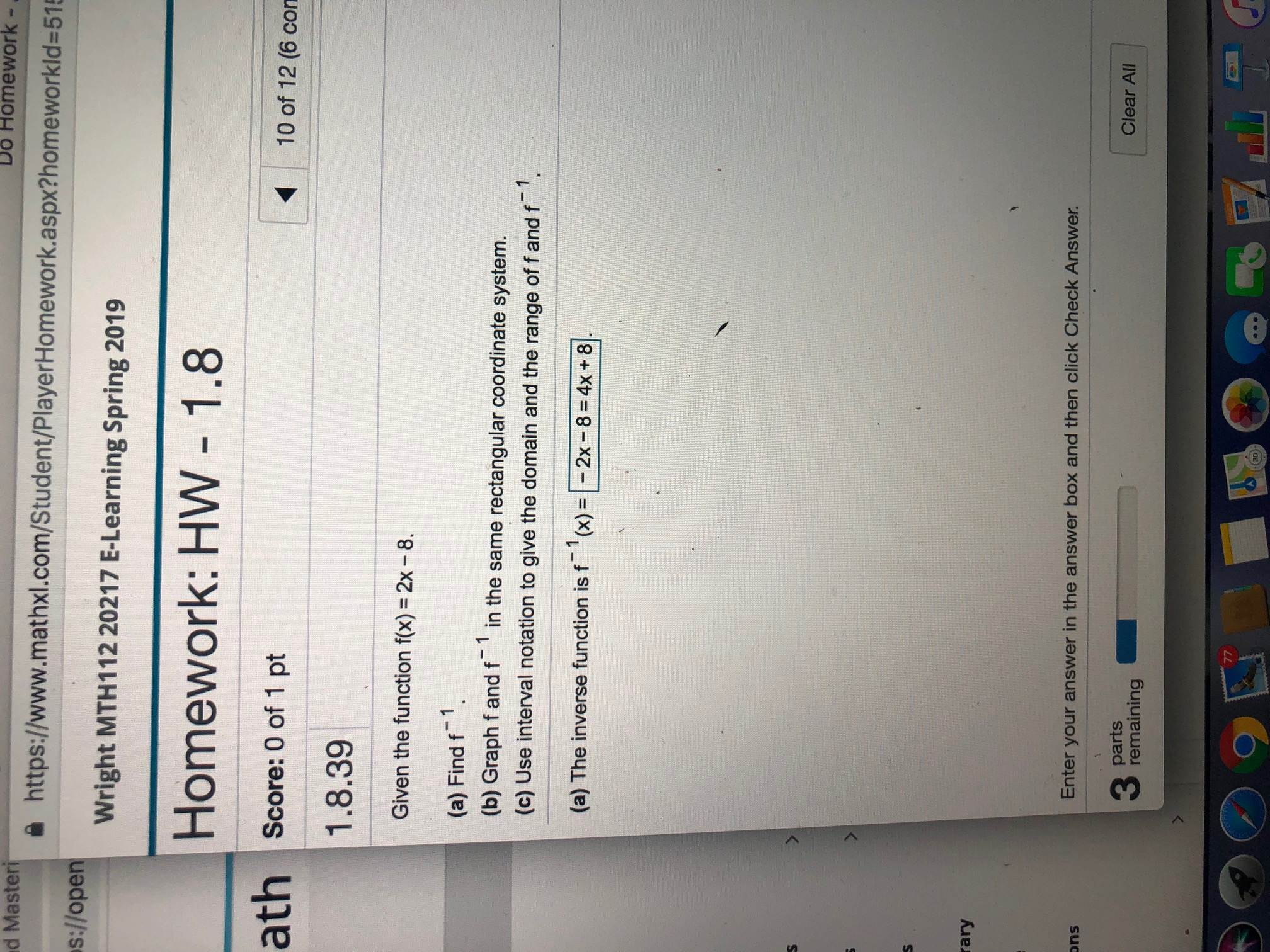# Do Homework -d Masteri욜 https://www.mathxl.com/Student/PlayerHomework.aspx?homeworkid=5%s:!lopen wrightWright MTH112 20217 E-Learning Spring 2019Homework: HW 1.8Score: 0 of 1 pt10 of 12 (6 con1.8.39Given the function f(x) = 2x-8.(a) Find f1.(b) Graph f and f in the same rectangular coordinate system.(c) Use interval notation to give the domain and the range of f and f(a) The inverse function is f-1 (x) = E 2x-8 = 4x + 81].raryEnter your answer in the answer box and then click Check Answer.onspartsClear All3 Pemtaining30

Question
1 viewshelp_outlineImage TranscriptioncloseDo Homework - d Masteri 욜 https://www.mathxl.com/Student/PlayerHomework.aspx?homeworkid=5% s:!lopen wright Wright MTH112 20217 E-Learning Spring 2019 Homework: HW 1.8 Score: 0 of 1 pt 10 of 12 (6 con 1.8.39 Given the function f(x) = 2x-8. (a) Find f1. (b) Graph f and f in the same rectangular coordinate system. (c) Use interval notation to give the domain and the range of f and f (a) The inverse function is f-1 (x) = E 2x-8 = 4x + 81 ]. rary Enter your answer in the answer box and then click Check Answer. ons parts Clear All 3 Pemtaining 30 fullscreen
check_circle

Step 1

(a) to find an explicit expression for the inverse (function) of f; (b) to plot the graphs of both f and its inverse; (c) to determine the domain and range of these functions using (b)

Step 2

In general, the inverse function (f^(-1)) of f is defined as in the diagram

Step 3

Following this definition , write y=f(x), solve for x and replace y b...

### Want to see the full answer?

See Solution

#### Want to see this answer and more?

Solutions are written by subject experts who are available 24/7. Questions are typically answered within 1 hour.*

See Solution
*Response times may vary by subject and question.
Tagged in

### Calculus